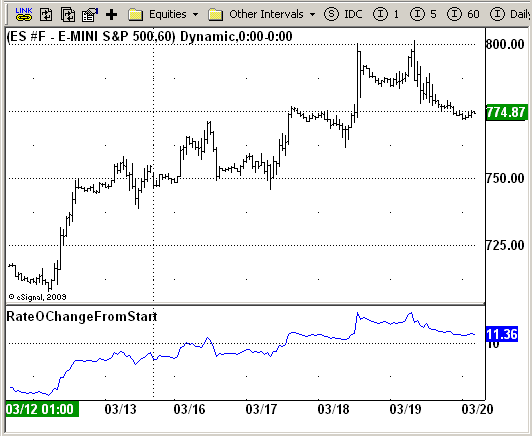# The Price Rate-Of-Change (R.O.C.) indicator

ICE Data Services -

RateOChangeFromStart.efs
EFSLibrary - Discussion Board

File Name: RateOChangeFromStart.efs

Description:
The Price Rate-Of-Change (R.O.C.) indicator.

Formula Parameters:

• Price Data To Use : Close

Notes:
Calculates the rate of change of the current Chosen Price as compared to the first price of data. this indicator is a modification of classic Rate-of-change indicator. The Price Rate-Of-Change (R.O.C.) indicator
(percent method) is calculated by dividing the price change over the last x-periods by the closing price of the security x-periods ago. The result is the percentage that the security's price has changed in the
last x-periods.
If the security's price is higher today than x-periods ago, the R.O.C. will be a positive number. If the security's price is lower today than x-periods ago, the R.O.C. will be a negative number.

RateOChangeFromStart.efsEFS Code:

```/*********************************
Provided By:
eSignal (Copyright c eSignal), a division of Interactive Data
Formula Script (EFS) is for educational purposes only and may be
modified and saved under a new file name.  eSignal is not responsible
for the functionality once modified.  eSignal reserves the right
to modify and overwrite this EFS file with each new release.

Description:
The Price Rate-Of-Change (R.O.C.) indicator.

Version:            1.0  03/19/2009

Formula Parameters:                     Default:
Price Data To Use                   Close

Notes:
Calculates the rate of change of the current Chosen Price as compared
to the first price of data. this indicator is a modification of classic
Rate-of-change indicator. The Price Rate-Of-Change (R.O.C.) indicator
(percent method) is calculated by dividing the price change over the
last x-periods by the closing price of the security x-periods ago. The
result is the percentage that the security's price has changed in the
last x-periods.
If the security's price is higher today than x-periods ago, the R.O.C.
will be a positive number. If the security's price is lower today than
x-periods ago, the R.O.C. will be a negative number.

**********************************/

var fpArray = new Array();
var bInit = false;

function preMain() {
setPriceStudy(false);
setStudyTitle("RateOChangeFromStart");
setCursorLabelName("RateOChangeFromStart");

var x = 0;
fpArray[x] = new FunctionParameter("sPrice", FunctionParameter.STRING);
with(fpArray[x++]){
setName("Price Data To Use");
setDefault("close");
}
}

var FVal = null;
var xPrice = null;
function main(sPrice) {
var nBarState = getBarState();
var nPrice = 0;
if (nBarState == BARSTATE_ALLBARS) {
if (sPrice == null) sPrice = "close";
}
if (bInit == false) {
xPrice = eval(sPrice)();
}
nPrice = xPrice.getValue(0);
if (nPrice == null) return;
if (FVal == null) FVal = nPrice;
var nRateOChangeFromStart = (nPrice * 100) / FVal - 100;
return nRateOChangeFromStart;
}```